# Civil Engineering - UPSC Civil Service Exam Questions

36.

Consider the following statements related to Los Angeles Abrasion test on aggregates :
1. It evaluates hardness of source - rock
2. It has a coefficient of variation of about 30 percent
Which of the statements given above is/are correct ?

 A. 1 only B. 2 only C. Both 1 and 2 D. Neither 1 nor 2

Explanation:

No answer description available for this question. Let us discuss.

37.

A bar of uniform cross-section of 400 mm2 is loaded as shown in the figure. The stress at section 1-1 is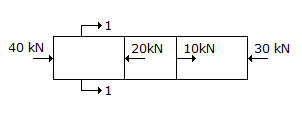A. 50 N/mm2 B. 100 N/mm2 C. 25 N/mm2 D. 200 N/mm2

Explanation:

No answer description available for this question. Let us discuss.

38.

Due to settlement of support at A of propped cantilever shown in the figure given below, what is the vertical reaction at B ?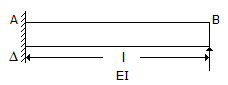A.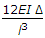B.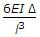C.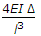D.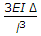Explanation:

No answer description available for this question. Let us discuss.

39.

A wooden plank (sp. gr. 0.5) 1 m x 1 m x 0.5 m floats in water with 1.5 kN load on it with 1 m x 1 m surface horizontal. The depth of plank lying below water surface shall be

 A. 0.178 m B. 0.250 m C. 0.403 m D. 0.500 m

Explanation:

No answer description available for this question. Let us discuss.

40.

A parabolic vertical curve is to be set out connecting two uniform grades of +0.6% and +.0%. The rate of change of grade is to be 0.06% per 30 m. The length of the curve will be

 A. 66 2/3 m B. 133 1/3 m C. 200 M D. 266 2/3 m